# Frank Solutions for Class 10 Maths Chapter 22 Heights and Distances

Frank Solutions for Class 10 Maths Chapter 22 Heights and Distances are the best study materials for those students who have difficulties in solving problems. Students can download the PDF of Frank Solutions for Class 10 Maths Chapter 22 from the accessible links. Frank Solutions include all the questions provided in the textbook that is prescribed for Class 10, in accordance with the ICSE Board.

Chapter 22 – Heights and Distances explains that height is the measurement of an object in the vertical direction, and distance is the measurement of an object from a particular point in the horizontal direction. This chapter deals with some special techniques which help in solving problems easily and quickly. These are basic identities which we use in higher studies.

## Download the PDF of Frank Solutions for Class 10 Maths Chapter 22 Heights and Distances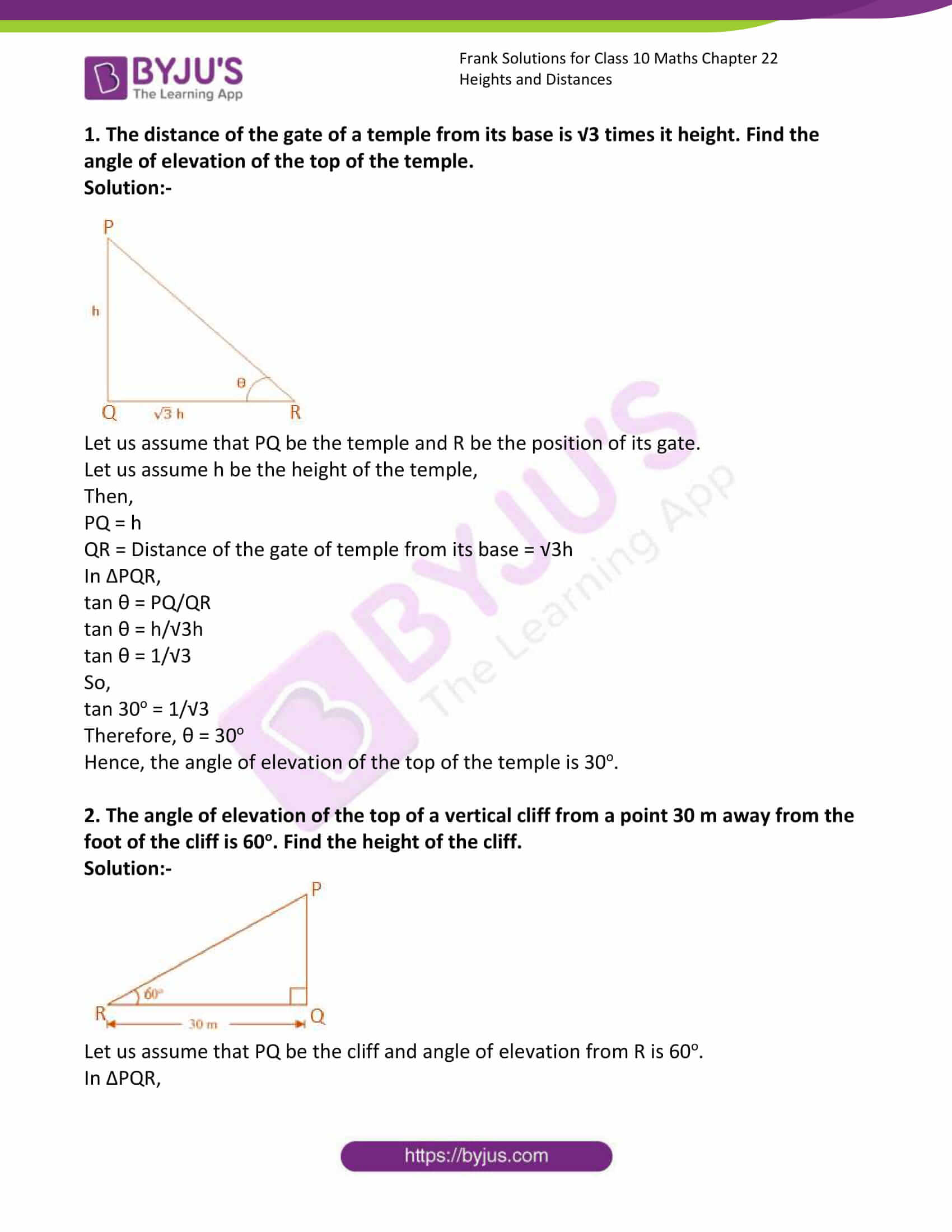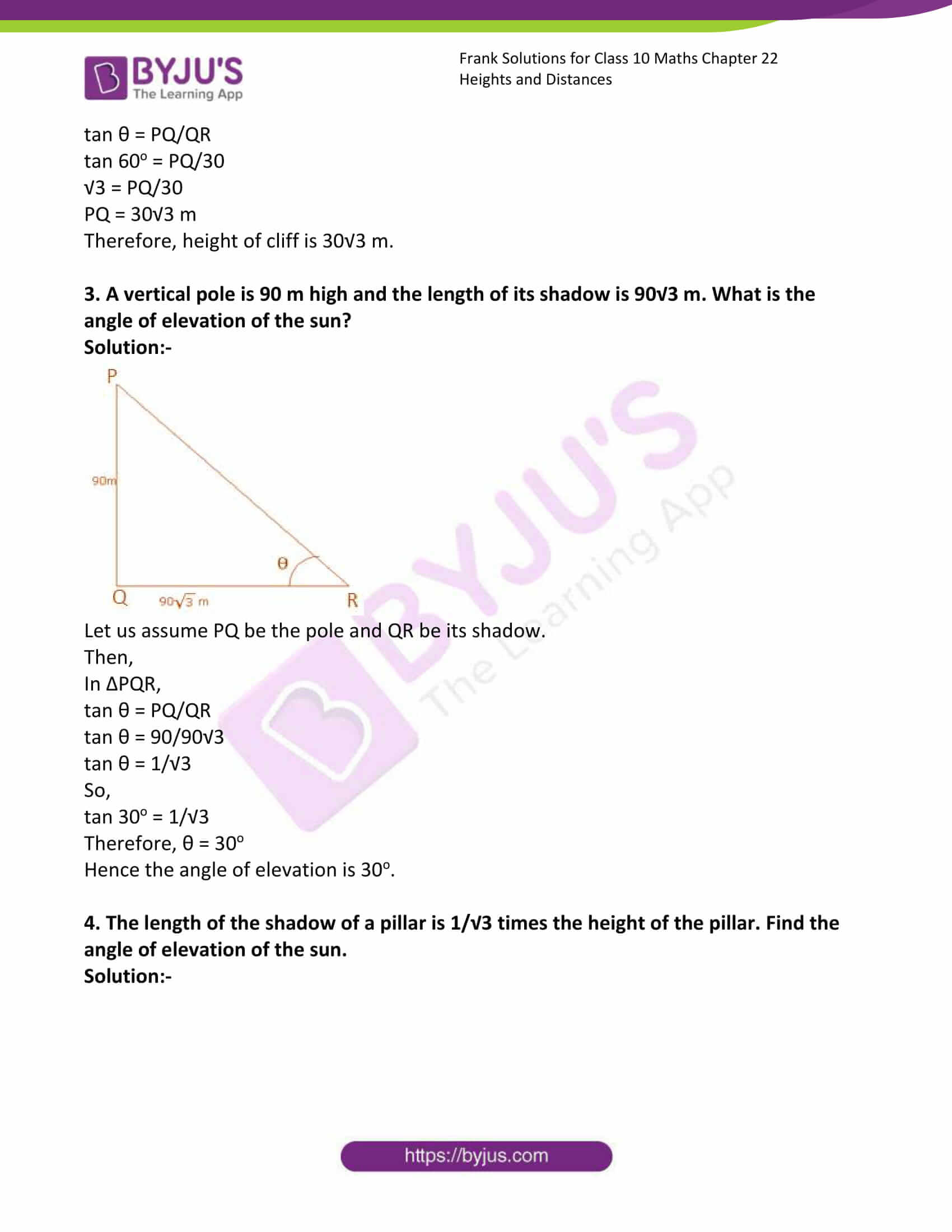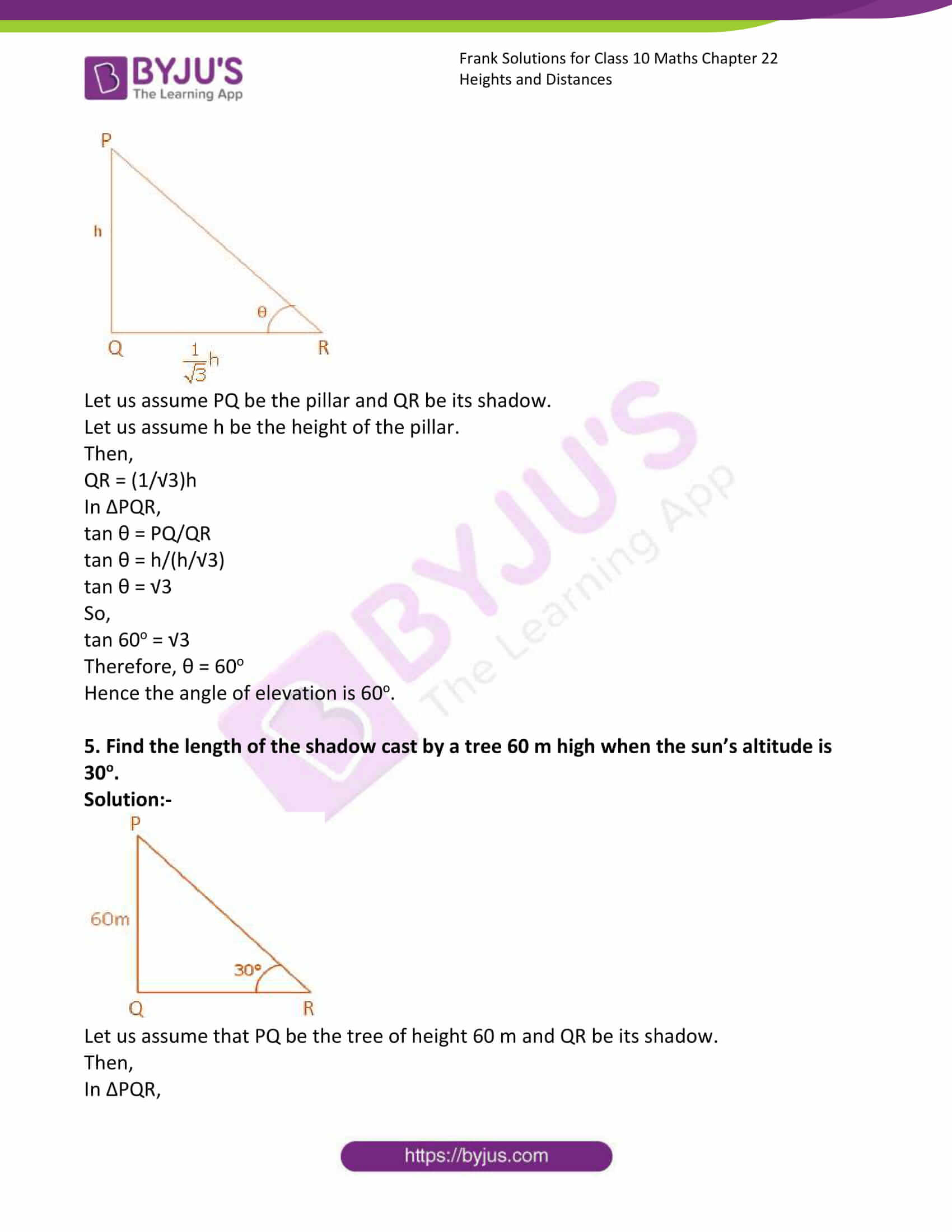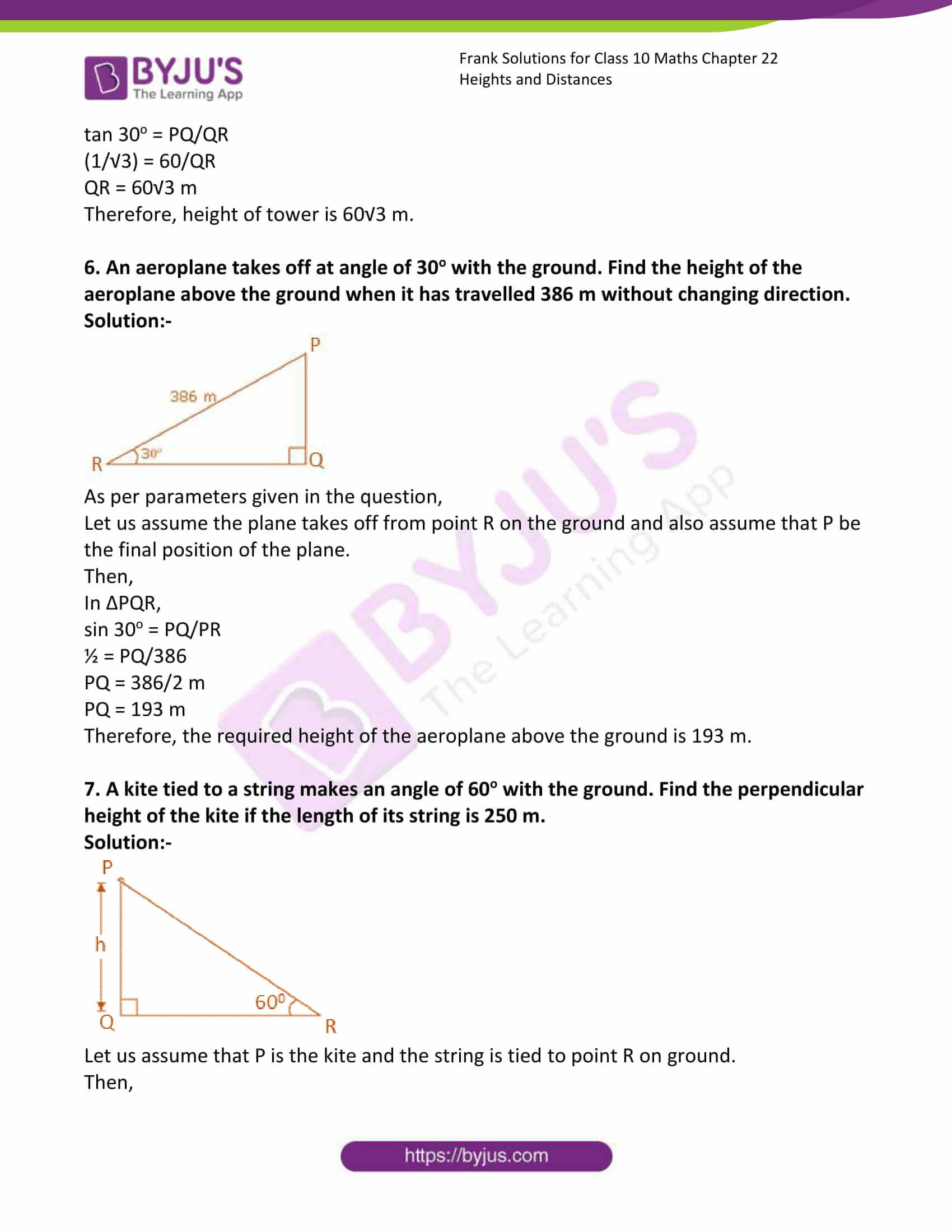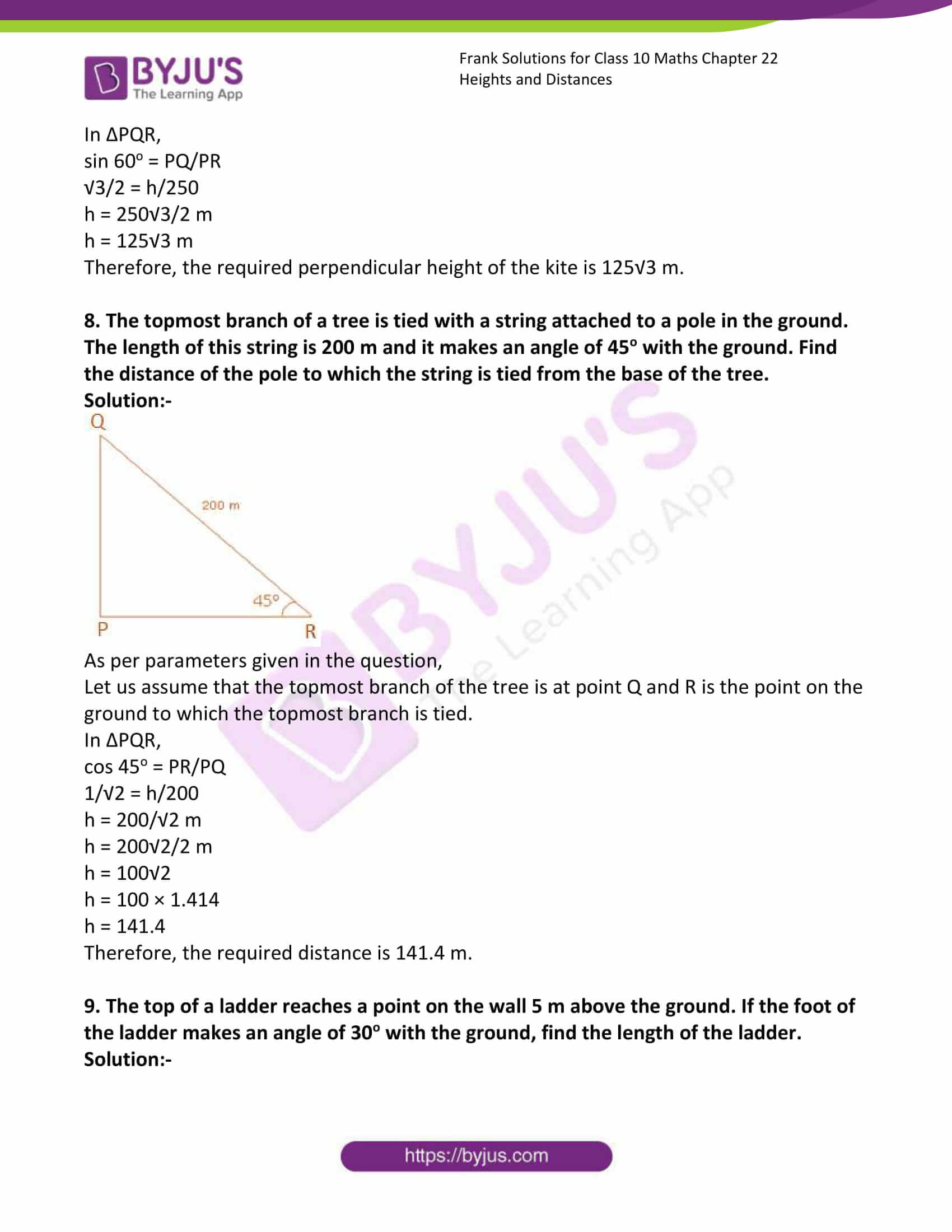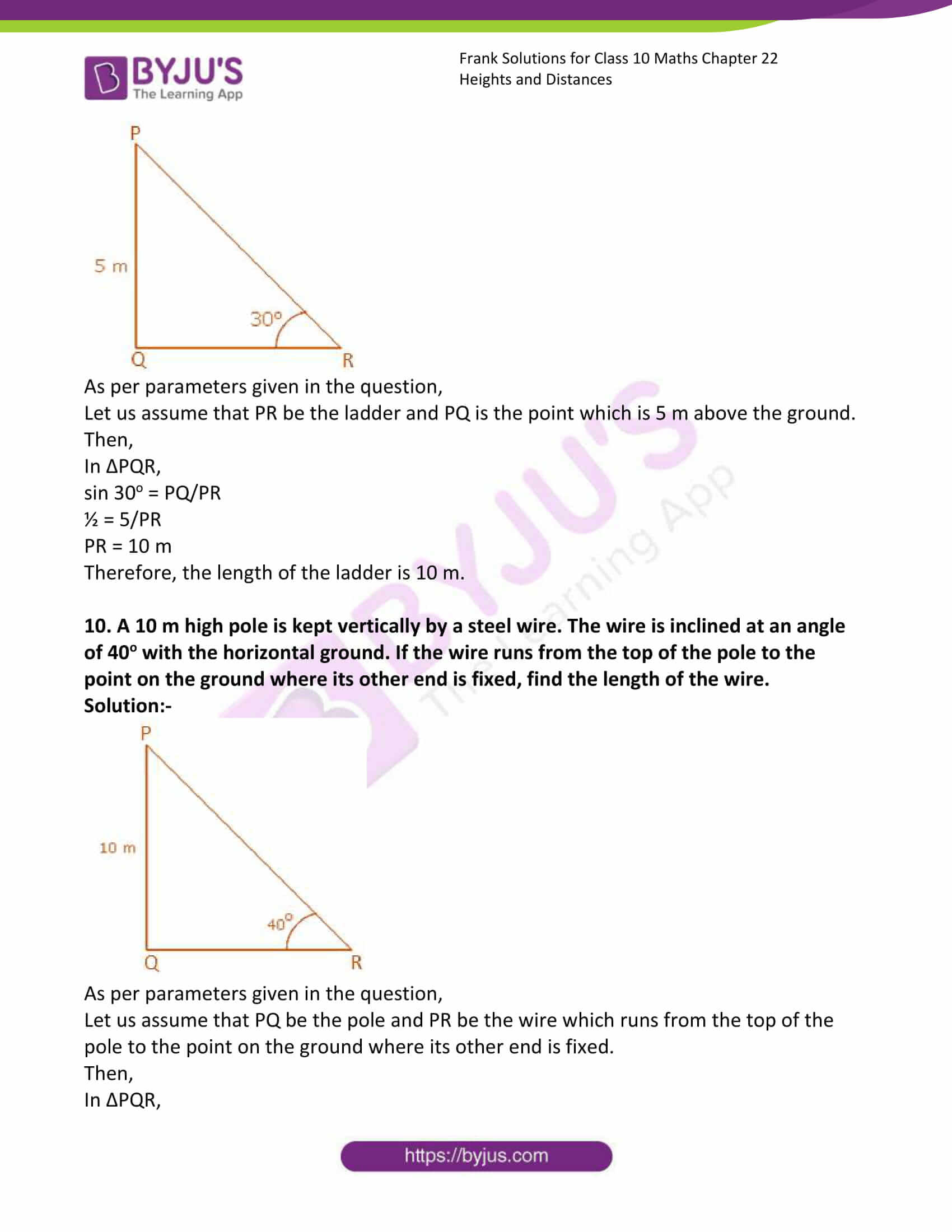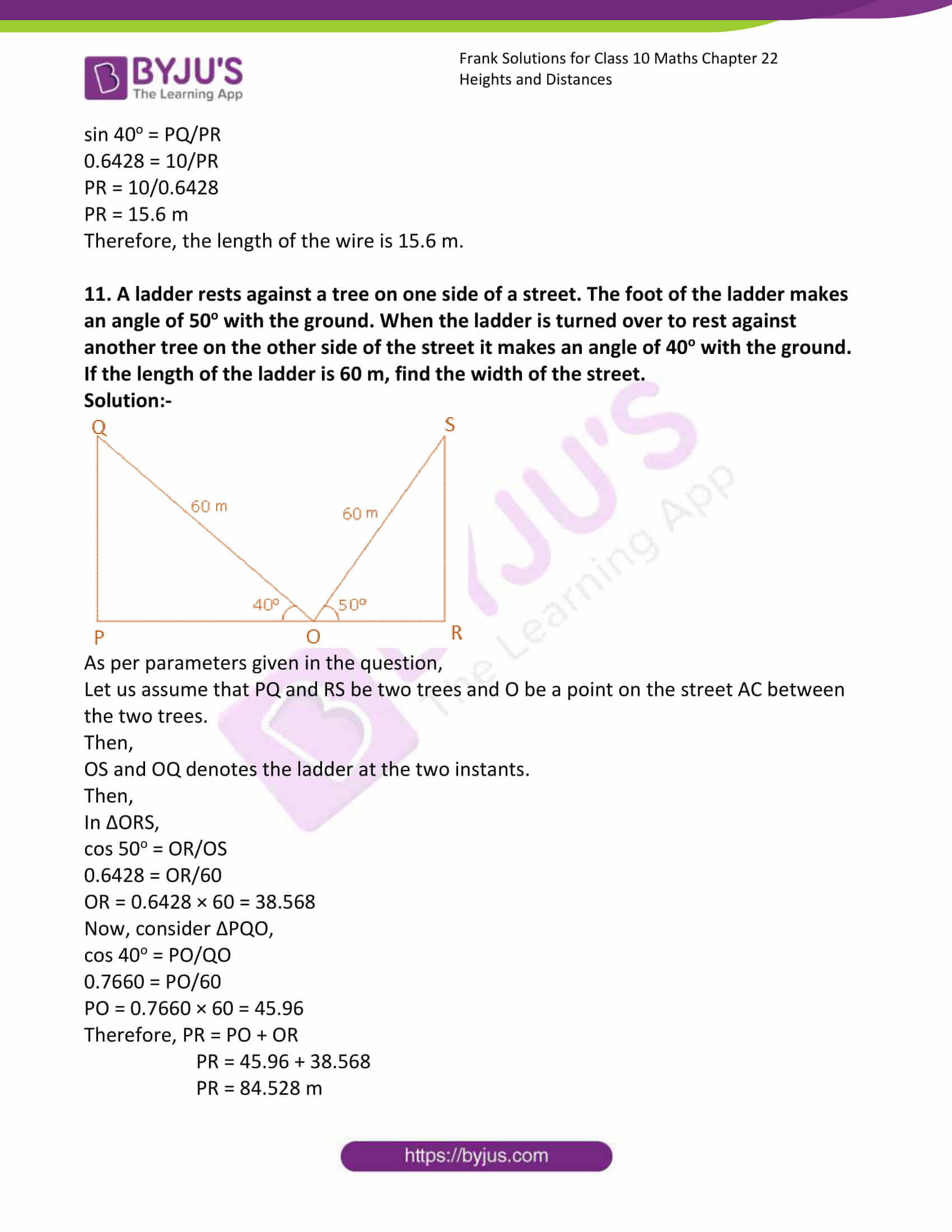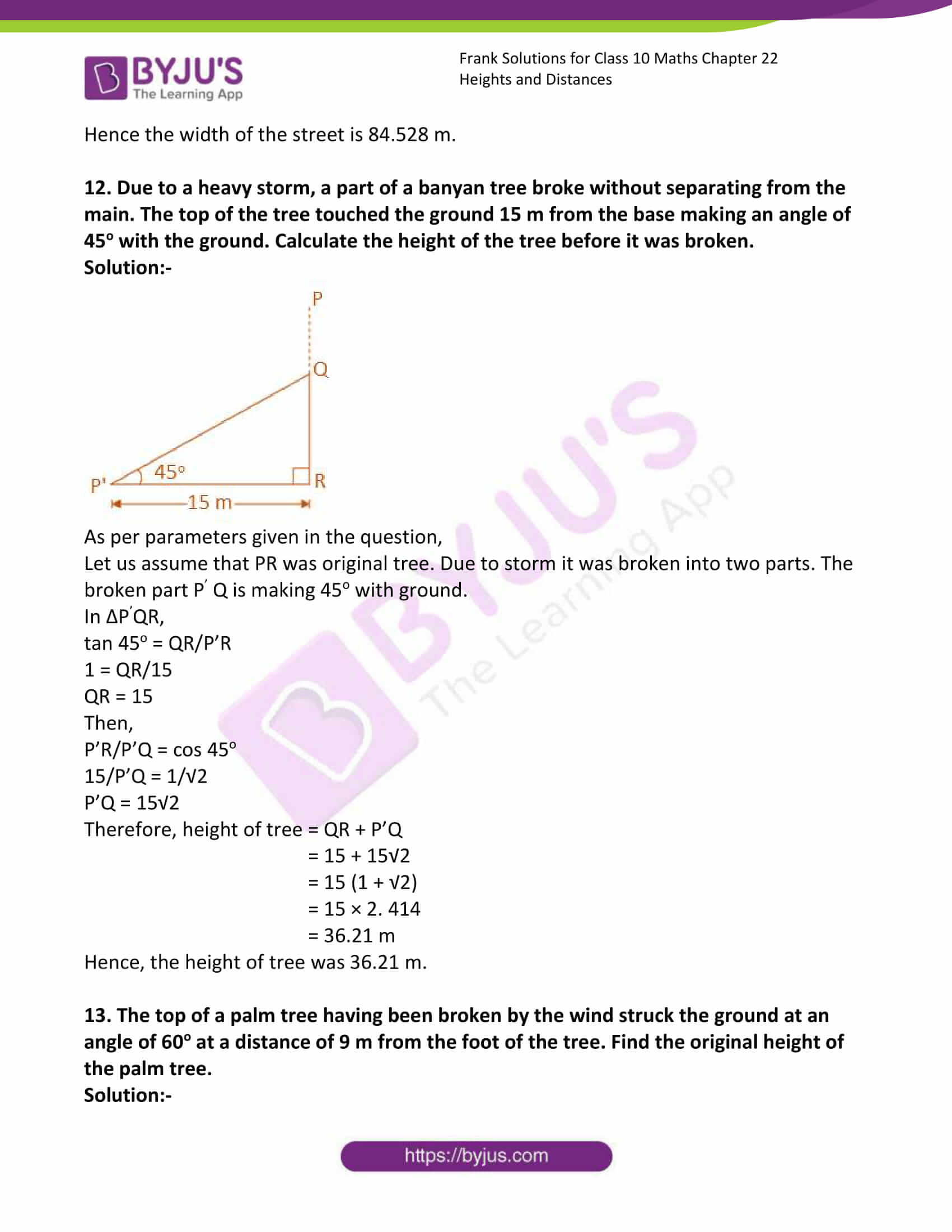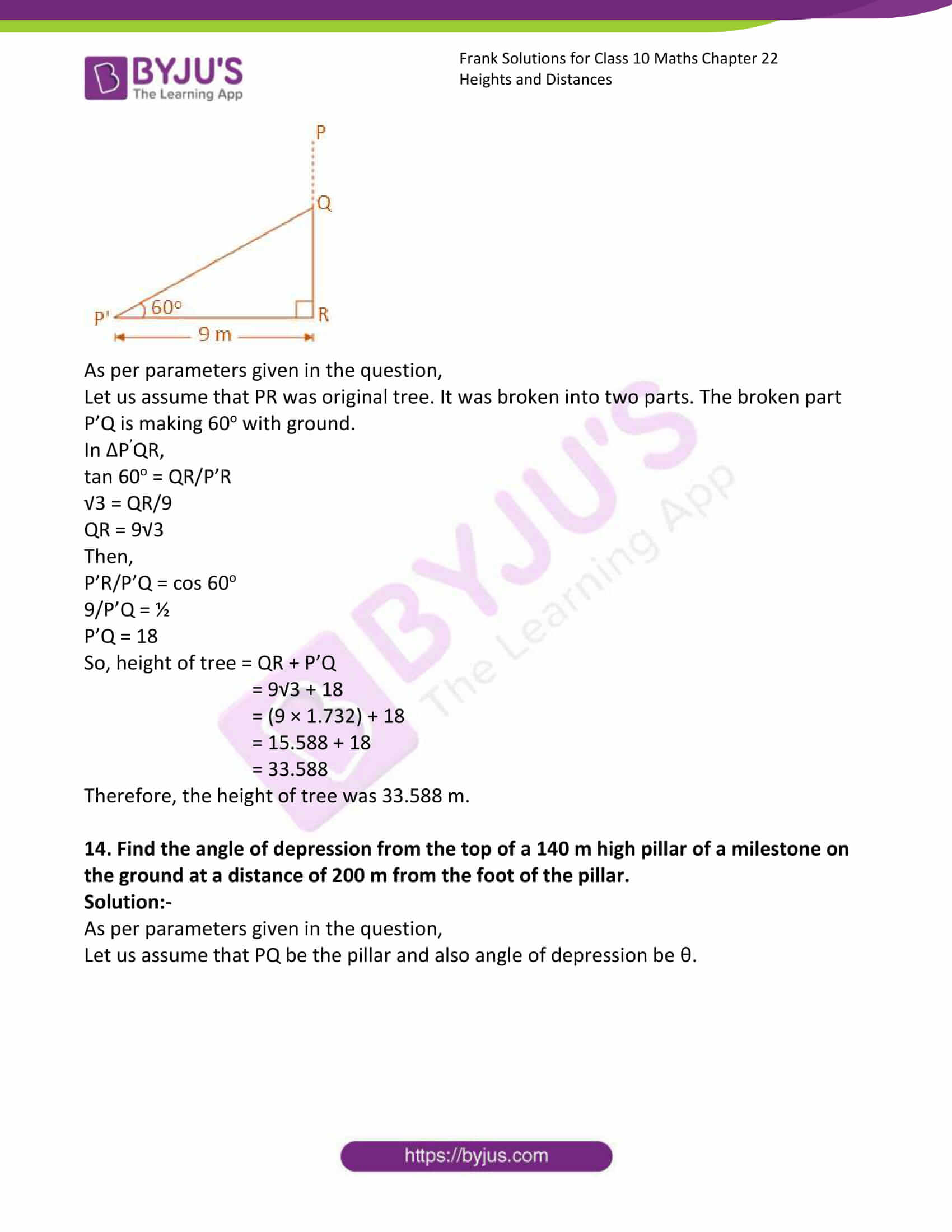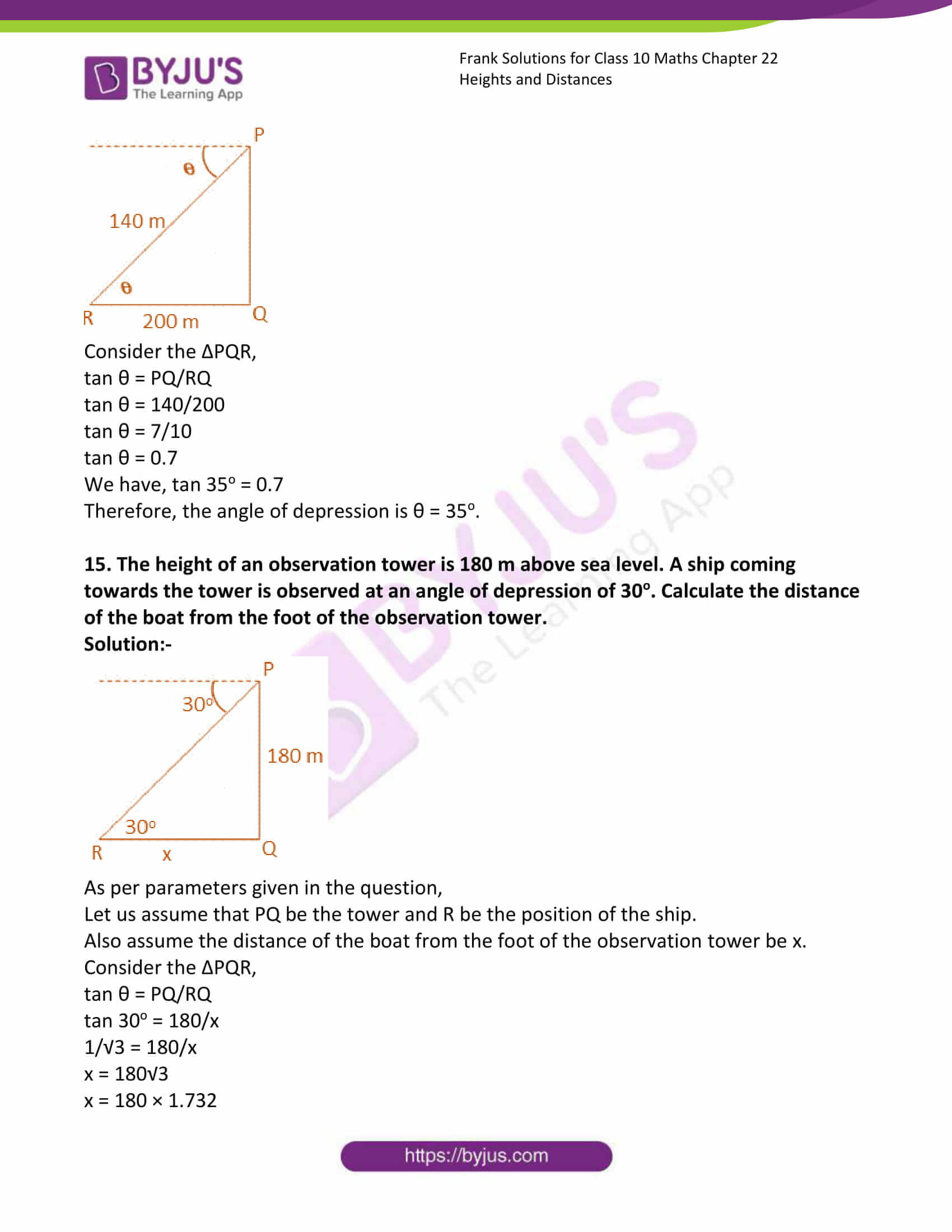### Access Answers to Frank Solutions for Class 10 Maths Chapter 22 Heights and Distances

1. The distance of the gate of a temple from its base is √3 times it height. Find the angle of elevation of the top of the temple.

Solution:-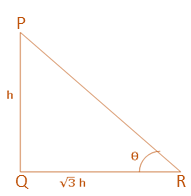Let us assume that PQ be the temple and R be the position of its gate.

Let us assume h be the height of the temple,

Then,

PQ = h

QR = Distance of the gate of temple from its base = √3h

In ΔPQR,

tan θ = PQ/QR

tan θ = h/√3h

tan θ = 1/√3

So,

tan 30o = 1/√3

Therefore, θ = 30o

Hence, the angle of elevation of the top of the temple is 30o.

2. The angle of elevation of the top of a vertical cliff from a point 30 m away from the foot of the cliff is 60o. Find the height of the cliff.

Solution:-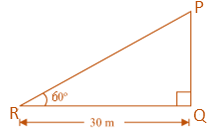Let us assume that PQ be the cliff and angle of elevation from R is 60o.

In ΔPQR,

tan θ = PQ/QR

tan 60o = PQ/30

√3 = PQ/30

PQ = 30√3 m

Therefore, height of cliff is 30√3 m.

3. A vertical pole is 90 m high and the length of its shadow is 90√3 m. What is the angle of elevation of the sun?

Solution:-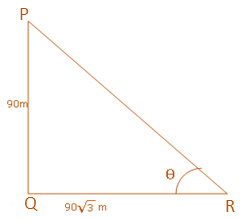Let us assume PQ be the pole and QR be its shadow.

Then,

In ΔPQR,

tan θ = PQ/QR

tan θ = 90/90√3

tan θ = 1/√3

So,

tan 30o = 1/√3

Therefore, θ = 30o

Hence the angle of elevation is 30o.

4. The length of the shadow of a pillar is 1/√3 times the height of the pillar. Find the angle of elevation of the sun.

Solution:-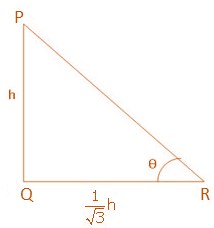Let us assume PQ be the pillar and QR be its shadow.

Let us assume h be the height of the pillar.

Then,

QR = (1/√3)h

In ΔPQR,

tan θ = PQ/QR

tan θ = h/(h/√3)

tan θ = √3

So,

tan 60o = √3

Therefore, θ = 60o

Hence the angle of elevation is 60o.

5. Find the length of the shadow cast by a tree 60 m high when the sun’s altitude is 30o.
Solution:-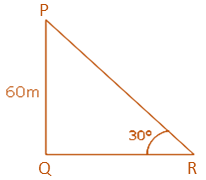Let us assume that PQ be the tree of height 60 m and QR be its shadow.

Then,

In ΔPQR,

tan 30o = PQ/QR

(1/√3) = 60/QR

QR = 60√3 m

Therefore, height of tower is 60√3 m.

6. An aeroplane takes off at angle of 30o with the ground. Find the height of the aeroplane above the ground when it has travelled 386 m without changing direction.

Solution:-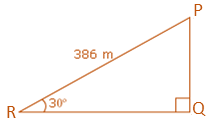As per parameters given in the question,

Let us assume the plane takes off from point R on the ground and also assume that P be the final position of the plane.

Then,

In ΔPQR,

sin 30o = PQ/PR

½ = PQ/386

PQ = 386/2 m

PQ = 193 m

Therefore, the required height of the aeroplane above the ground is 193 m.

7. A kite tied to a string makes an angle of 60o with the ground. Find the perpendicular height of the kite if the length of its string is 250 m.
Solution:-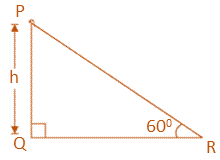Let us assume that P is the kite and the string is tied to point R on ground.

Then,

In ΔPQR,

sin 60o = PQ/PR

√3/2 = h/250

h = 250√3/2 m

h = 125√3 m

Therefore, the required perpendicular height of the kite is 125√3 m.

8. The topmost branch of a tree is tied with a string attached to a pole in the ground. The length of this string is 200 m and it makes an angle of 45o with the ground. Find the distance of the pole to which the string is tied from the base of the tree.

Solution:-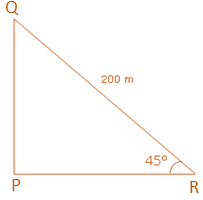As per parameters given in the question,

Let us assume that the topmost branch of the tree is at point Q and R is the point on the ground to which the topmost branch is tied.

In ΔPQR,

cos 45o = PR/PQ

1/√2 = h/200

h = 200/√2 m

h = 200√2/2 m

h = 100√2

h = 100 × 1.414

h = 141.4

Therefore, the required distance is 141.4 m.

9. The top of a ladder reaches a point on the wall 5 m above the ground. If the foot of the ladder makes an angle of 30o with the ground, find the length of the ladder.

Solution:-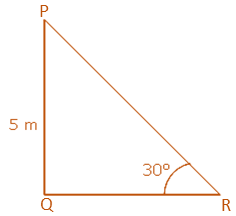As per parameters given in the question,

Let us assume that PR be the ladder and PQ is the point which is 5 m above the ground.

Then,

In ΔPQR,

sin 30o = PQ/PR

½ = 5/PR

PR = 10 m

Therefore, the length of the ladder is 10 m.

10. A 10 m high pole is kept vertically by a steel wire. The wire is inclined at an angle of 40o with the horizontal ground. If the wire runs from the top of the pole to the point on the ground where its other end is fixed, find the length of the wire.

Solution:-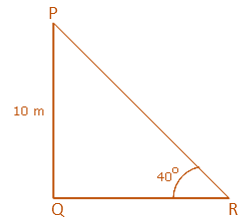As per parameters given in the question,

Let us assume that PQ be the pole and PR be the wire which runs from the top of the pole to the point on the ground where its other end is fixed.

Then,

In ΔPQR,

sin 40o = PQ/PR

0.6428 = 10/PR

PR = 10/0.6428

PR = 15.6 m

Therefore, the length of the wire is 15.6 m.

11. A ladder rests against a tree on one side of a street. The foot of the ladder makes an angle of 50o with the ground. When the ladder is turned over to rest against another tree on the other side of the street it makes an angle of 40o with the ground. If the length of the ladder is 60 m, find the width of the street.

Solution:-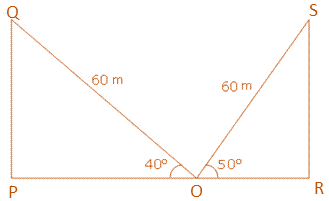As per parameters given in the question,

Let us assume that PQ and RS be two trees and O be a point on the street AC between the two trees.

Then,

OS and OQ denotes the ladder at the two instants.

Then,

In ΔORS,

cos 50o = OR/OS

0.6428 = OR/60

OR = 0.6428 × 60 = 38.568

Now, consider ΔPQO,

cos 40o = PO/QO

0.7660 = PO/60

PO = 0.7660 × 60 = 45.96

Therefore, PR = PO + OR

PR = 45.96 + 38.568

PR = 84.528 m

Hence the width of the street is 84.528 m.

12. Due to a heavy storm, a part of a banyan tree broke without separating from the main. The top of the tree touched the ground 15 m from the base making an angle of 45o with the ground. Calculate the height of the tree before it was broken.

Solution:-As per parameters given in the question,

Let us assume that PR was original tree. Due to storm it was broken into two parts. The broken part P Q is making 45o with ground.

In ΔPQR,

tan 45o = QR/P’R

1 = QR/15

QR = 15

Then,

P’R/P’Q = cos 45o

15/P’Q = 1/√2

P’Q = 15√2

Therefore, height of tree = QR + P’Q

= 15 + 15√2

= 15 (1 + √2)

= 15 × 2. 414

= 36.21 m

Hence, the height of tree was 36.21 m.

13. The top of a palm tree having been broken by the wind struck the ground at an angle of 60o at a distance of 9 m from the foot of the tree. Find the original height of the palm tree.

Solution:-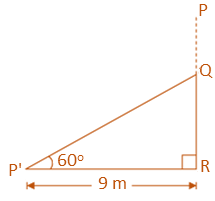As per parameters given in the question,

Let us assume that PR was original tree. It was broken into two parts. The broken part P’Q is making 60o with ground.

In ΔPQR,

tan 60o = QR/P’R

√3 = QR/9

QR = 9√3

Then,

P’R/P’Q = cos 60o

9/P’Q = ½

P’Q = 18

So, height of tree = QR + P’Q

= 9√3 + 18

= (9 × 1.732) + 18

= 15.588 + 18

= 33.588

Therefore, the height of tree was 33.588 m.

14. Find the angle of depression from the top of a 140 m high pillar of a milestone on the ground at a distance of 200 m from the foot of the pillar.

Solution:-

As per parameters given in the question,

Let us assume that PQ be the pillar and also angle of depression be θ.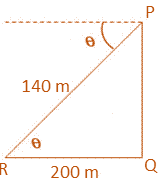Consider the ΔPQR,

tan θ = PQ/RQ

tan θ = 140/200

tan θ = 7/10

tan θ = 0.7

We have, tan 35o = 0.7

Therefore, the angle of depression is θ = 35o.

15. The height of an observation tower is 180 m above sea level. A ship coming towards the tower is observed at an angle of depression of 30o. Calculate the distance of the boat from the foot of the observation tower.

Solution:-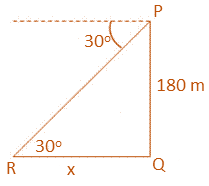As per parameters given in the question,

Let us assume that PQ be the tower and R be the position of the ship.

Also assume the distance of the boat from the foot of the observation tower be x.

Consider the ΔPQR,

tan θ = PQ/RQ

tan 30o = 180/x

1/√3 = 180/x

x = 180√3

x = 180 × 1.732

x = 311.76

Therefore, the distance of the boat from the foot of the observation tower is 311.76 m.